# 机器学习|感知机和人工神经网络详解

## 感知机

### 感知机的推导过程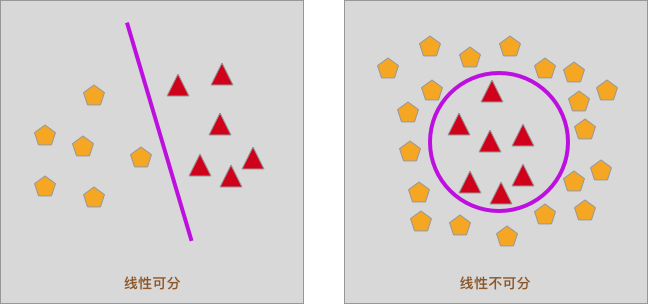$$f(x) = w_1x_1+w_2x_2+ \cdots +w_nx_n + b = WX+b \tag{1}$$

$$sign(x) = \begin{cases} +1, & \text{if } x \geq 0 \\ -1, & \text{if } x < 0 \end{cases} \tag{2}$$

sign() 函数又被称之为符号函数，它的函数值只有 2 个。即当自变量 $x≥0$ 时，因变量为 1。同理，当 $x<0$ 时，因变量为 -1。函数图像如下：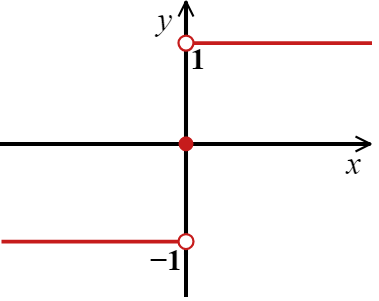$$f(x) = sign(w*x +b) \tag{3}$$

### 感知机计算流程图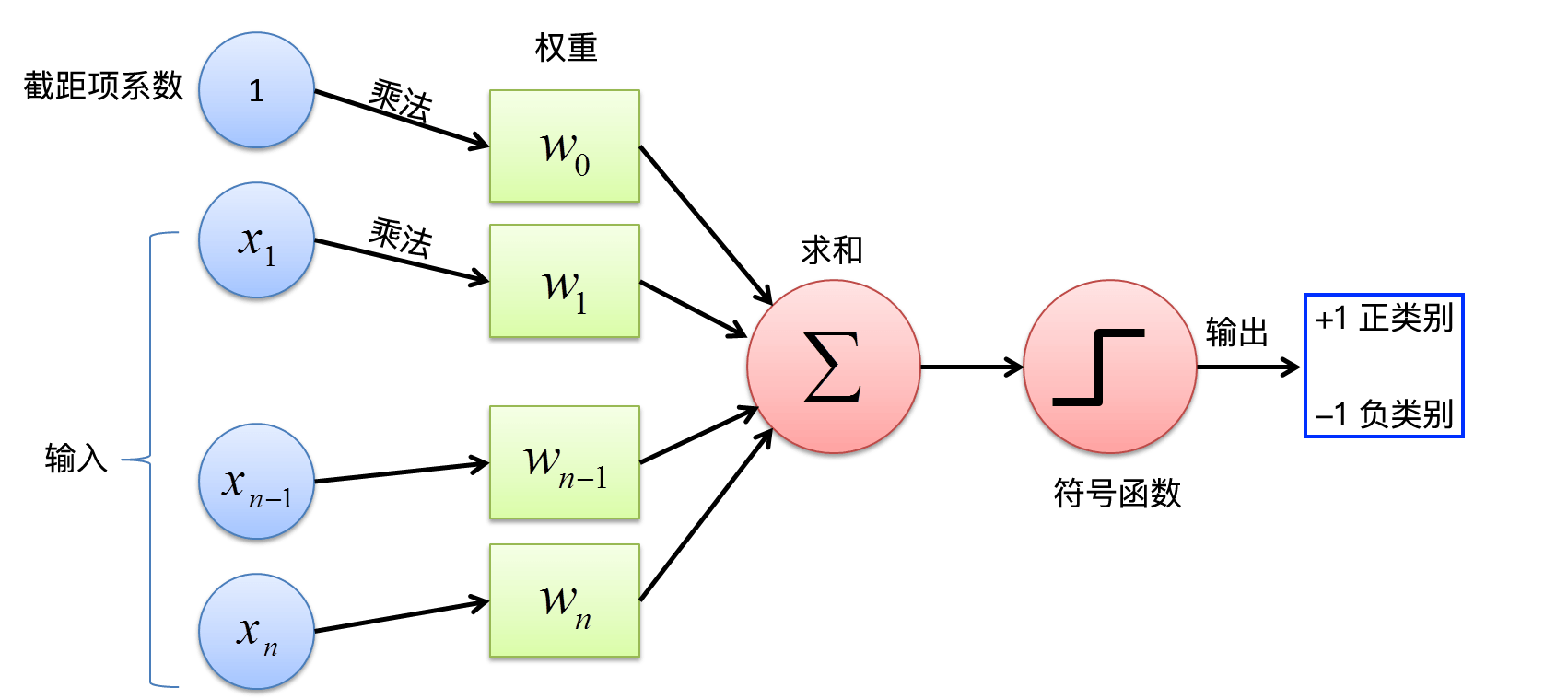### 感知机的损失函数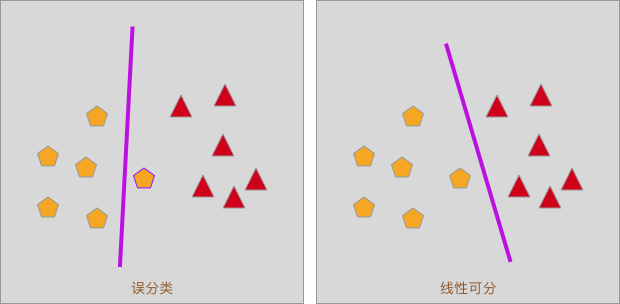#### 点到直线的距离

$$d= \dfrac{1}{\parallel W\parallel}|W*x_{0}+b| \tag{4}$$

$$y_i(W * x_{i}+b)>0 \tag{5}$$

$$d=-\dfrac{1}{\parallel W\parallel}y_i(W*x_{i}+b) \tag{6}$$

$$-\dfrac{1}{\parallel W\parallel}\sum_{x_i\epsilon M} y_i(W*x_{i}+b) \tag{7}$$

$$J(W,b) = - \sum_{x_i\epsilon M} y_i(W*x_{i}+b) \tag{8}$$

### 随机梯度下降法

$$min_{W,b} J(W,b) = - \sum_{x_i\epsilon M} y_i(W*x_{i}+b) \tag{9}$$

$$\frac{\partial J(W,b)}{\partial W} = - \sum_{x_i\epsilon M}y_ix_i \ \frac{\partial J(W,b)}{\partial b} = - \sum_{x_i\epsilon M}y_i \tag{10}$$

$$W \leftarrow W + \lambda y_ix_i \ b \leftarrow b + \lambda y_i \tag{11}$$

### 感知机分类实例

#### 示例数据集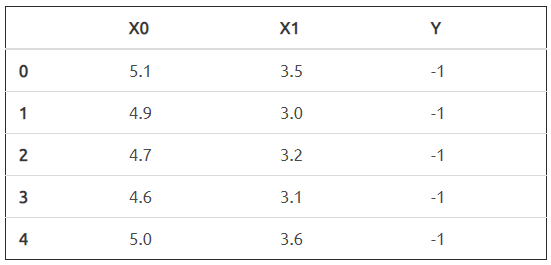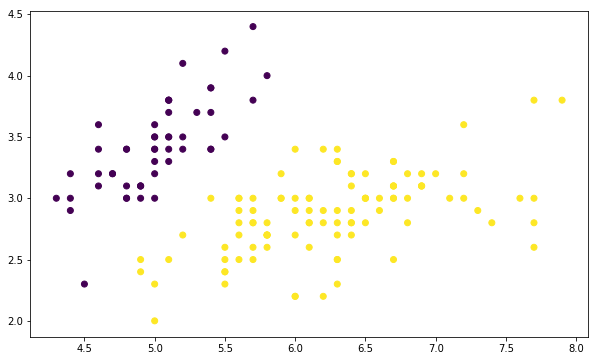#### 感知机训练

$$f(x) = 4.93 * x_{1} - 6.98 * x_{2} -3.3 \tag{12}$$

#### 绘制决策边界线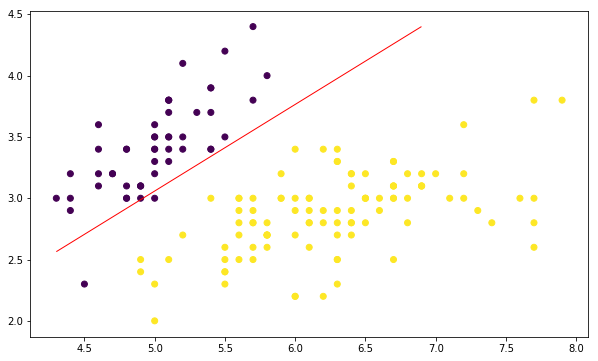#### 绘制损失函数变换曲线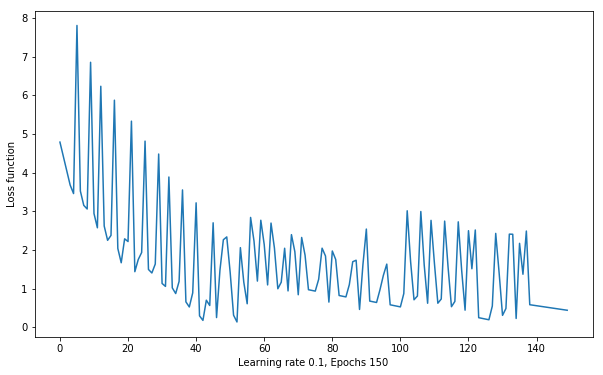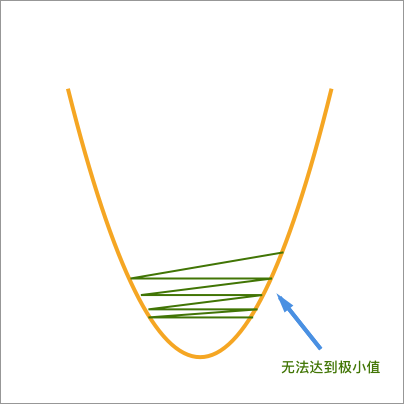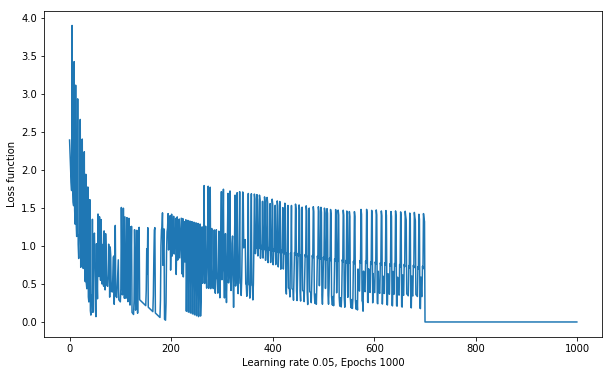## 人工神经网络

### 多层感知机与人工神经网络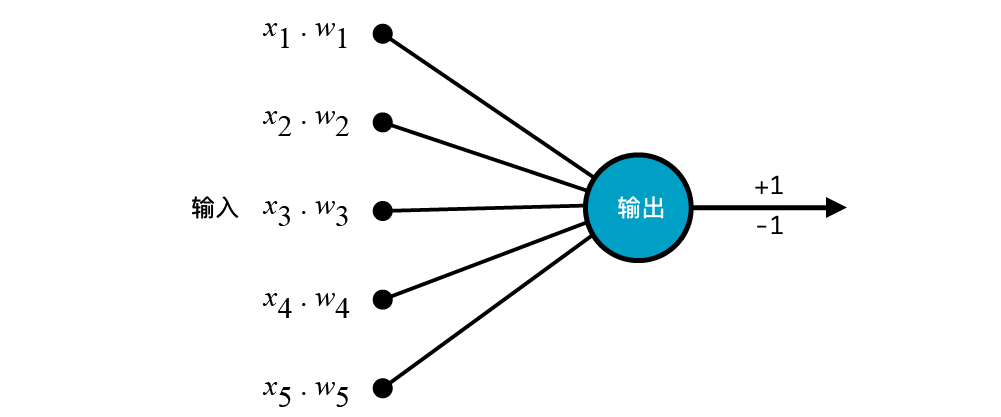### 激活函数

$$f(x) = w_1x_1+w_2x_2+ \cdots +w_nx_n + b = WX+b \tag{13}$$

• 对于逻辑回归而言，我们是采用了 $sigmoid$ 函数将 $f(x)$ 转换为概率，最终实现二分类。
• 对于感知机而言，我们是采用了 $sign$ 函数将 $f(x)$ 转换为 -1 和 +1 最终实现二分类。
• 对于多层感知机而言，具有多层神经网络结构，在 $f(x)$ 的处理方式上，一般会有更多的操作。

#### $sigmoid$ 函数

$sigmoid$ 函数应该已经非常熟悉了吧，它的公式如下：

$$sigmoid(x)=\frac{1}{1+e^{-x}} \tag{14}$$

$sigmoid$ 函数的图像呈 S 型，函数值介于 $(0,1)$ 之间：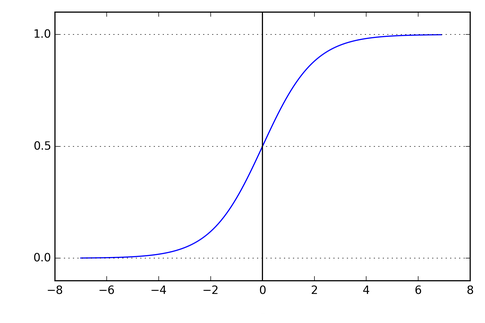#### $Tanh$ 函数

$Tanh$ 函数与 $sigmoid$ 函数的图像很相似，都呈 S 型，只不过 $Tanh$ 函数值介于 $(-1,1)$ 之间，公式如下：

$$tanh(x)=\frac{1-e^{-2x}}{1+e^{-2x}}\tag{15}$$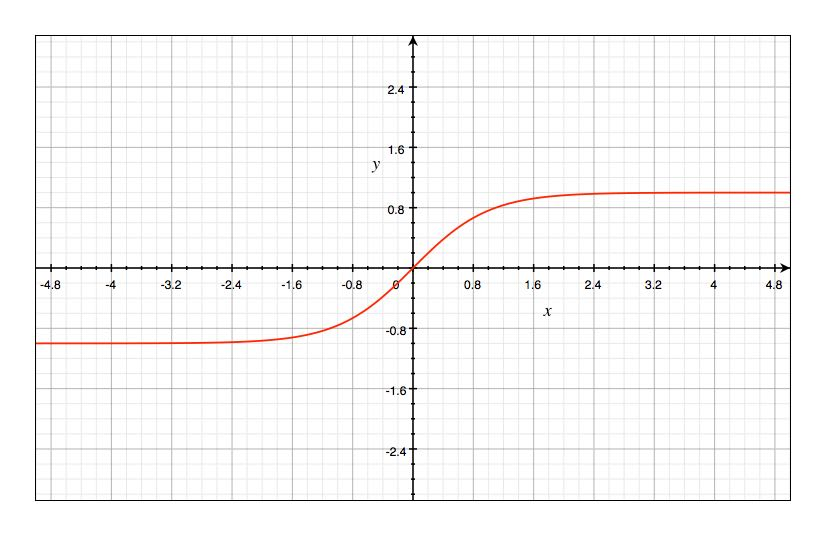#### $ReLU$ 函数

$ReLU$ 函数全称叫做 Rectified Linear Unit，也就是修正线性单元，公式如下：

$$ReLU(x) = max(0,x)\tag{16}$$

$ReLU$ 有很多优点，比如收敛速度会较快且不容易出现梯度消失。由于这次不会用到，我们之后再说。$ReLU$的图像如下：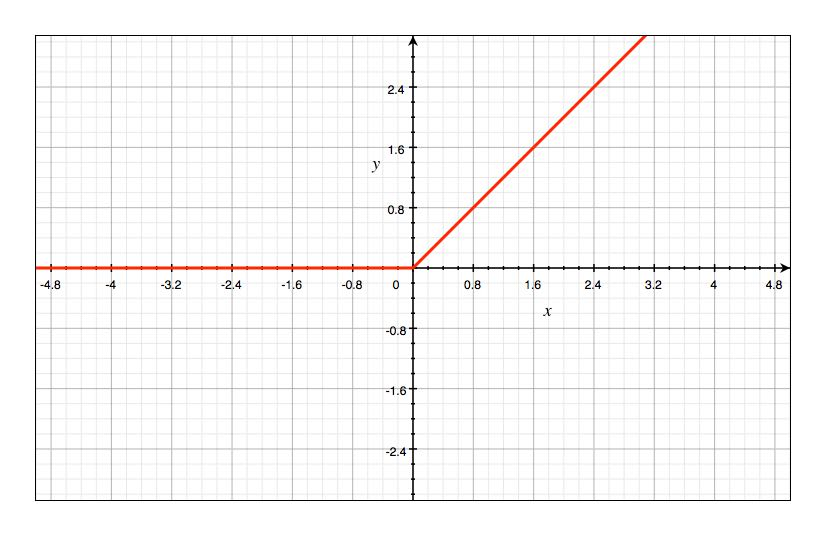#### 激活函数的作用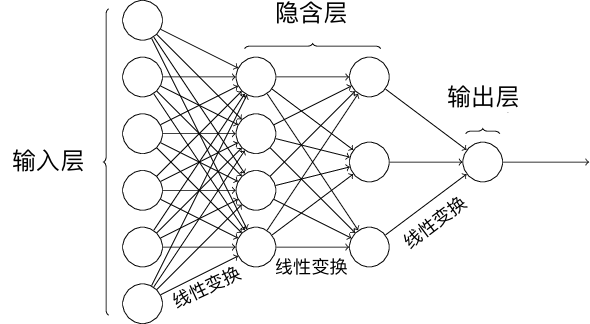### 反向传播算法（BP）直观认识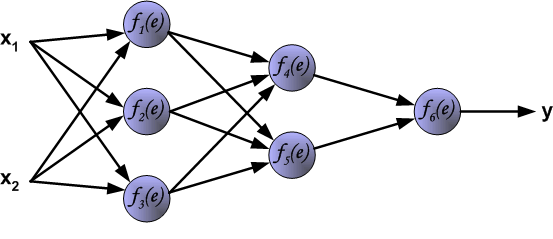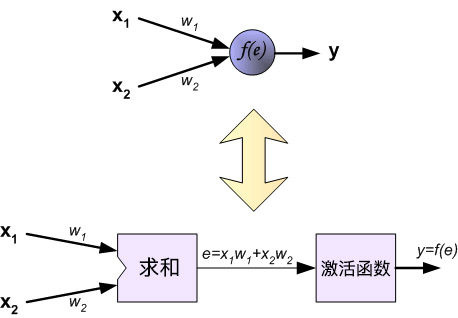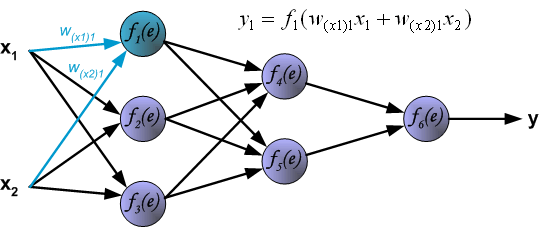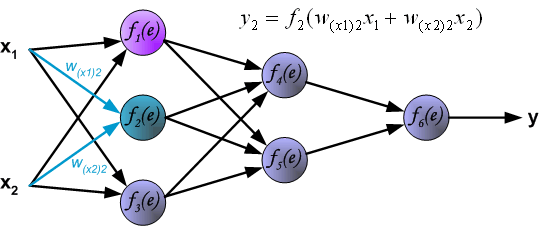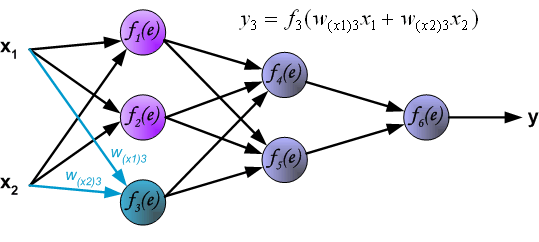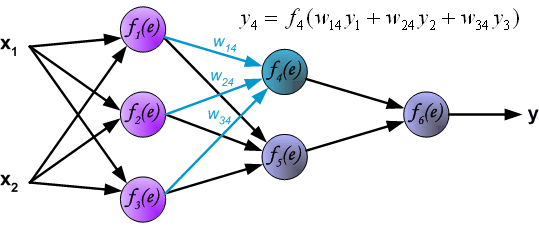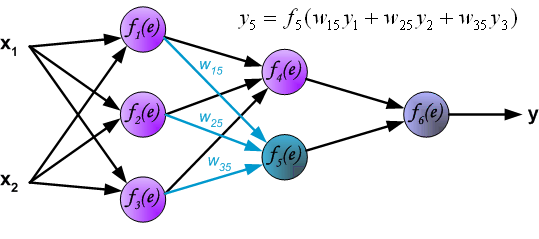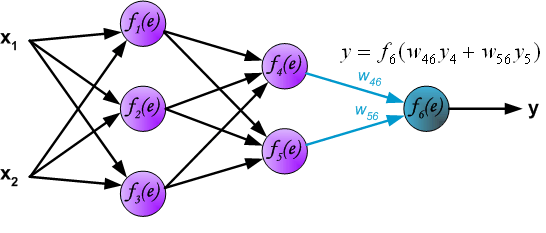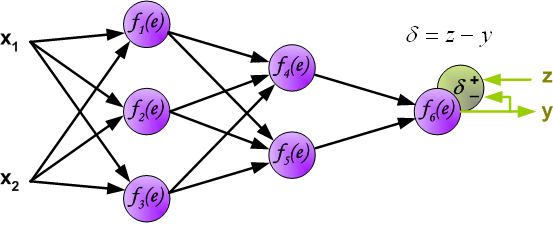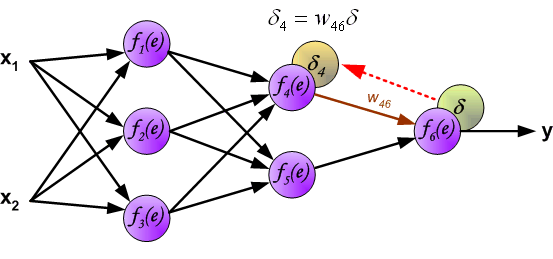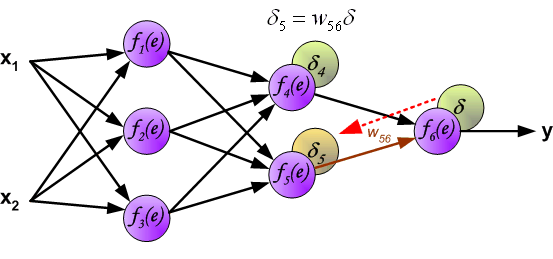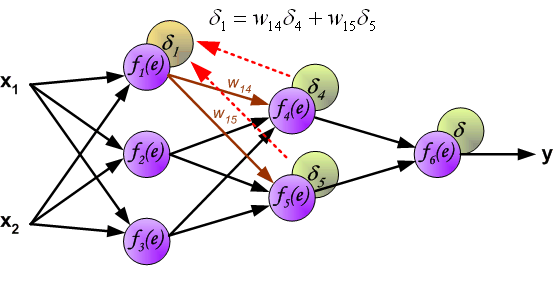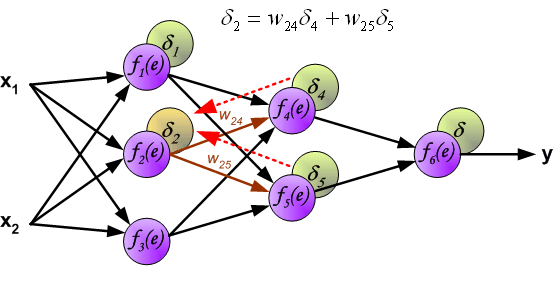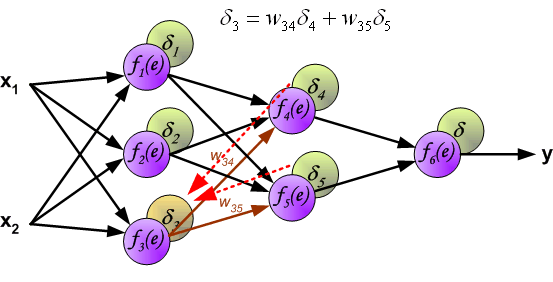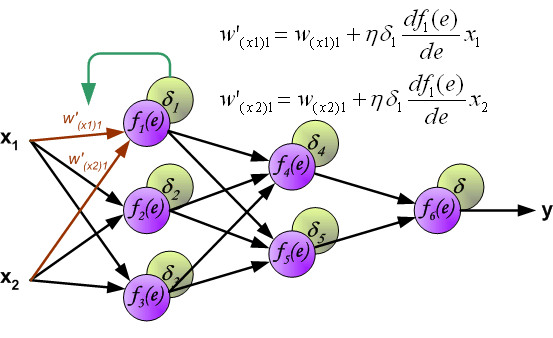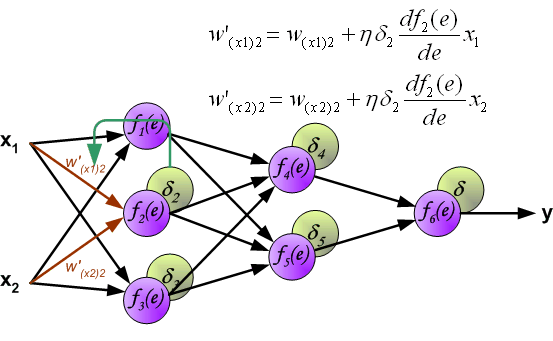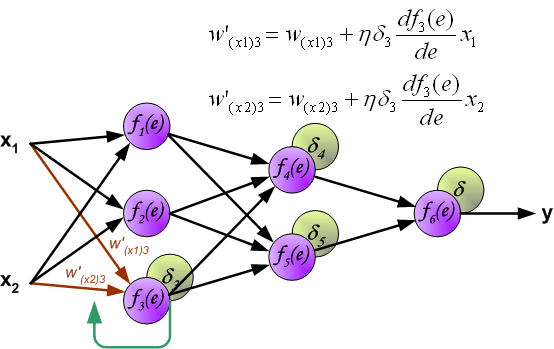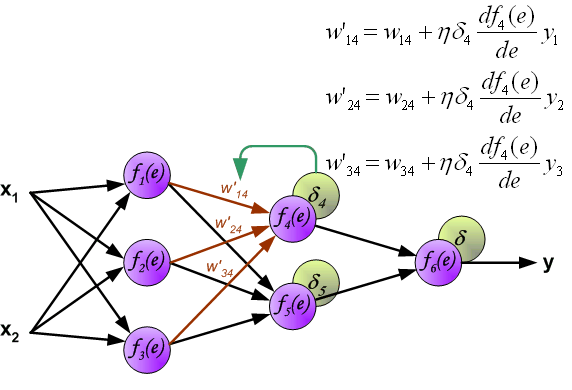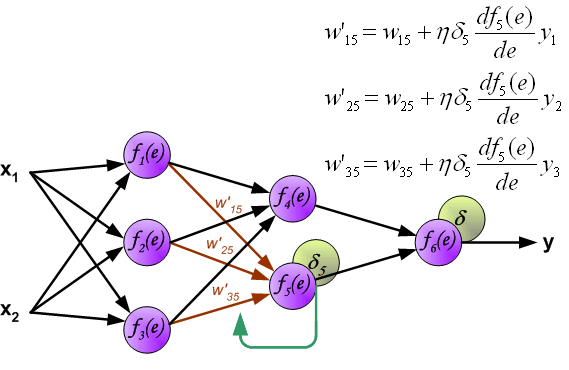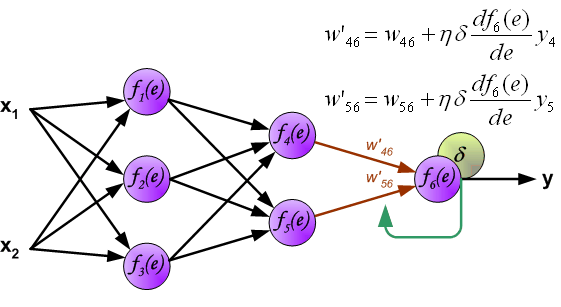### 使用 Python 实现人工神经网络

#### 定义神经网络结构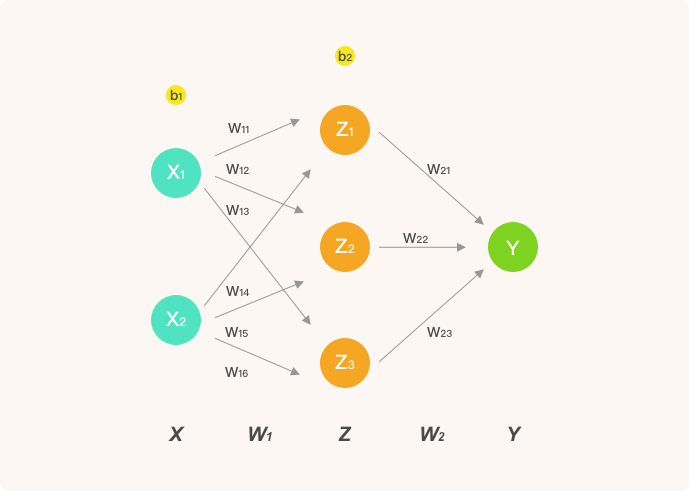$$\mathit{sigmoid}(x) = \frac{1}{1+e^{-x}} \tag{17a}$$

$$\Delta \mathit{sigmoid}(x) = \mathit{sigmoid}(x)(1 - \mathit{sigmoid}(x)) \tag{17b}$$

#### 前向传播

• $Z$ 表示隐含层输出，$Y$ 则为输出层最终输出。
• $w_ij$ 表示从第 $i$ 层的第 $j$ 个权重。

$$X = \begin{bmatrix} x_{1} & x_{2} \end{bmatrix} \tag{18}$$

$$W_1 = \begin{bmatrix} w_{11} & w_{12} & w_{13}\ w_{14} & w_{15} & w_{16}\ \end{bmatrix} \tag{19}$$

$$W_2 = \begin{bmatrix} w_{21} \ w_{22} \ w_{23} \end{bmatrix} \tag{20}$$

$$Z = \mathit{sigmoid}(X \cdot W_{1}) \tag{21}$$

$$Y = \mathit{sigmoid}(Z \cdot W_{2}) \tag{22}$$

#### 反向传播

$$Loss(y, Y) = \sum (y - Y)^2 \tag{23}$$

$$\frac{\partial Loss(y, Y)}{\partial{W_2}} = \frac{\partial Loss(y, Y)}{\partial{Y}} \frac{\partial Y}{\partial{W_2}}\tag{24a}$$

$$\frac{\partial Loss(y, Y)}{\partial{W_2}} = 2(Y-y) * \Delta \mathit{sigmoid}(Z \cdot W_2) \cdot Z\tag{24b}$$

$$\frac{\partial Loss(y, Y)}{\partial{W_1}} = \frac{\partial Loss(y, Y)}{\partial{Y}} \frac{\partial Y }{\partial{Z}} \frac{\partial Z}{\partial{W_1}} \tag{25a}$$

$$\frac{\partial Loss(y, Y)}{\partial{W_1}} = 2(Y-y) * \Delta \mathit{sigmoid}(Z \cdot W_2) \cdot W_2 * \Delta \mathit{sigmoid}(X \cdot W_1) \cdot X \tag{25b}$$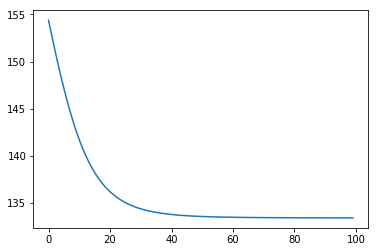http://blog.laugh12321.cn/2019/01/31/neural_network/

laugh12321

2019-01-31

2020-10-23